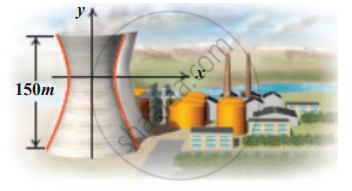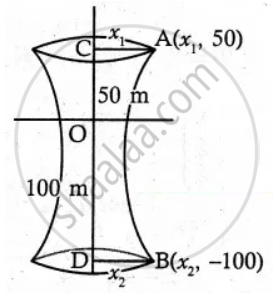Tamil Nadu Board of Secondary EducationHSC Arts Class 12th

# Cross-section of a Nuclear cooling tower is in the shape of a hyperbola with equation x2302-y2442 = 1. The tower is 150 m tall and the distance from the top of the tower to the centre of the hyperb - Mathematics

Sum

Cross-section of a Nuclear cooling tower is in the shape of a hyperbola with equation x^2/30^2 - y^2/44^2 = 1. The tower is 150 m tall and the distance from the top of the tower to the centre of the hyperbola is half the distance from the base of the tower to the centre of the hyperbola. Find the diameter of the top and base of the tower#### SolutionEquation of hyperbola is x^2/30^2 - y^2/44^2 = 1

Given OC = 1/2 OD and CD = 150

∴ OC = 50 m and OD = 100 m

Let the Radius of top of the tower be x1 and bottom of the tower be x2.

∴ Points A(x1, 50) and B(x2, 100)

Hyperbola passes through A(x1, 50)

∴ x^2/30^2 - 50^2/44^2 = 1

x_1^2/30^2 = 1 + 50^2/44^2

= 1 + 2500/1936

= (1936 + 2500)/1936

x_1^2 = 30/44 xx sqrt(4436)

⇒ x_1 = 30/44 xx sqrt(4436)

⇒ x_1 = 30/44 xx (66.6)

x1 = 45.41 m

∴ Radius of the top = 45.41 m.

Diameter of the top = 90.82 m

Also

The hyperbola again passes through B(x2, 100)

x^2/30^2 - 100^2/44^2 = 1

x_1^2/30^2 = 1 + 100^2/44^2

= (44^2 + 100^2)/44^2

x_2^2 = 30^2/44^2 (11936)

x_2 = 30/44 xx sqrt(11936)

= 30/44 (109.25)

x2 = 74.48

∴ Radius of the base = 74.48 m.

Diameter of the base = 148.96 m

∴ Diameter of the top and base of the tower are 90.82 m and 148.96 m

Concept: Real Life Applications of Conics
Is there an error in this question or solution?

#### APPEARS IN

Tamil Nadu Board Samacheer Kalvi Class 12th Mathematics Volume 1 and 2 Answers Guide
Chapter 5 Two Dimensional Analytical Geometry-II
Exercise 5.5 | Q 6 | Page 214
Share